# OECD Material productivity data analysis 1 - Using R to load CSV file data and reshape dataframe format with pivot_wider.Photo by Ivana Cajina on Unsplash

In this blog, I will analyze OECD Material productivity data.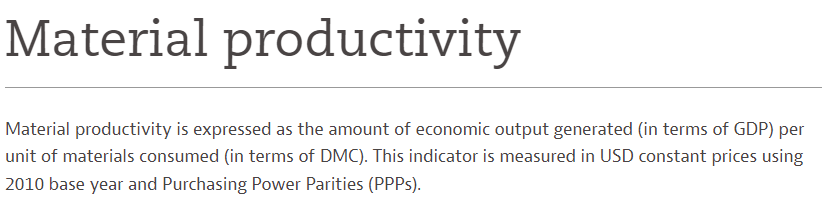First, I downloaded data from the OECD webiste: Materials - Material productivity - OECD Data

OECD (2022), Material productivity (indicator). doi: 10.1787/dae52b45-en (Accessed on 12 February 2022)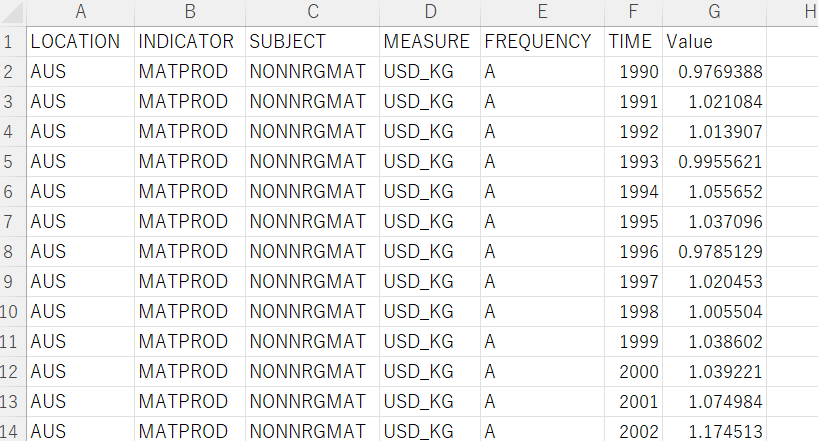I use R to analyze the data, so I firstly load tidyverse package and use read_csv() function to load the CSV file.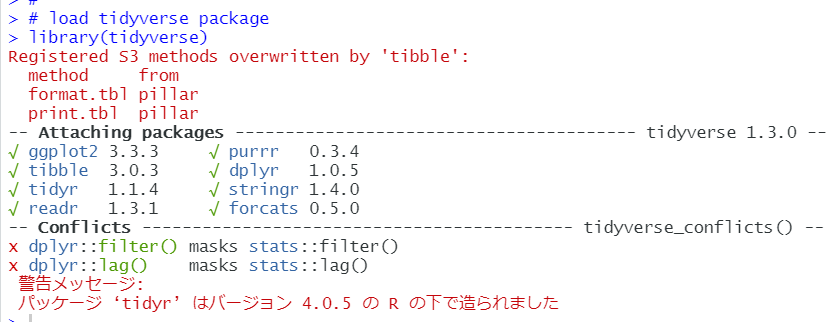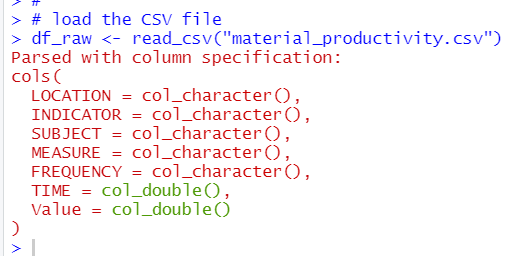Let's check each variables. For character variables, how many vales are there, for numeric variables, summary statistics.

LOCATION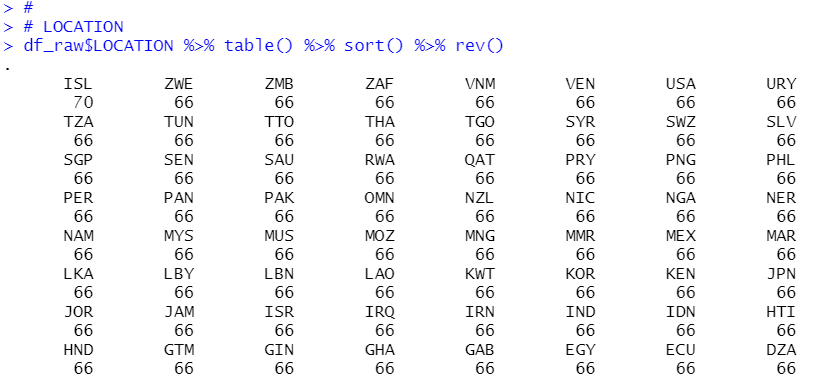There are many locations.

INDICATOR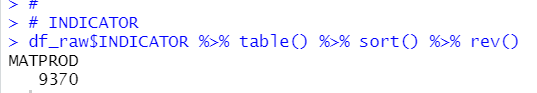For INDICATOR, there is only one value, MATPROD, so we can delete INDICATOR.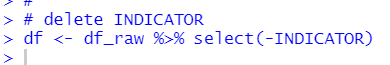SUBJECT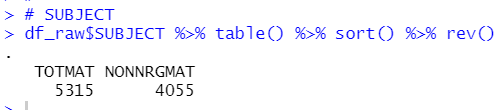There are two kinds of vakue, TOTMAT and NONNRGMAT. So I keep SUBJECT.

MEASURE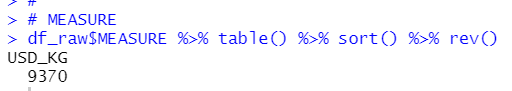There is only one value in MEASURE, it is USD_KG. I will remove MEASURE.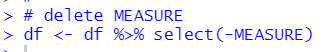FREQUENCY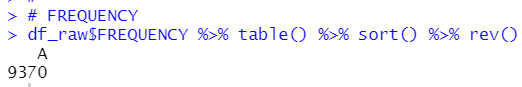There is only one value in FRQUENCY, it is A. I will remove FREQUENCY.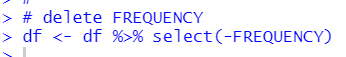TIME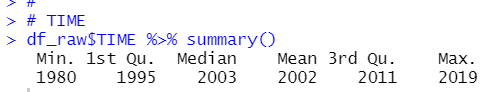For TIME, it starts in 1980 and ends in 2019. There is not NA value.

Value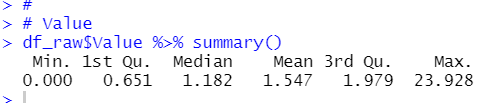There is not NA value in Value.
Now, my new dataframe, df has LOCATION, SUBJECT, TIME and Value.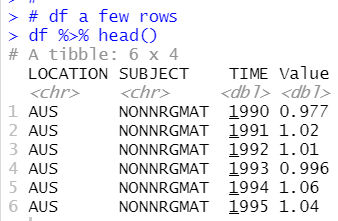I know SUBJECT has two kinds of value, one is TOTMAT and the oter is NONNRGMAT.
So, I want to reshape my dataframe df like below

LOCATION TIME TOTMAT NONNRGMAT
AUS            1990  XXX       XXX
AUS            1991  XXX       XXX
AUS            1992  XXX       XXX

So, I will use pivot_wider() function.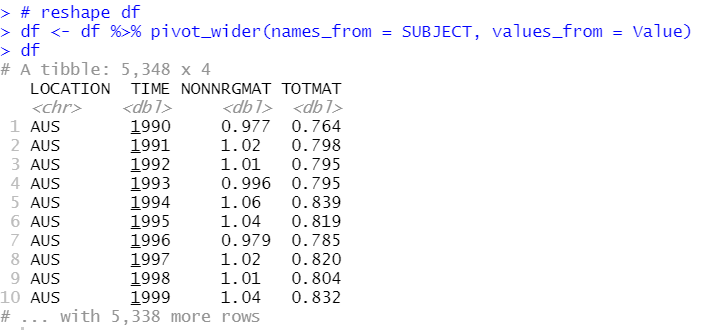All right.
Let's check summary statistics.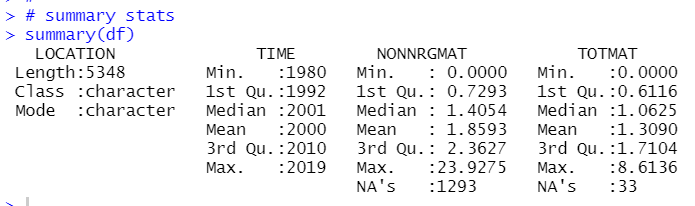There are several NA's in NONNRGMAT and TOTMAT. So, I will delete NA rows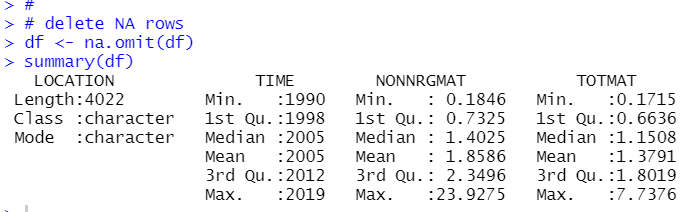By the way, I have GDP and per capita GDP data file like below.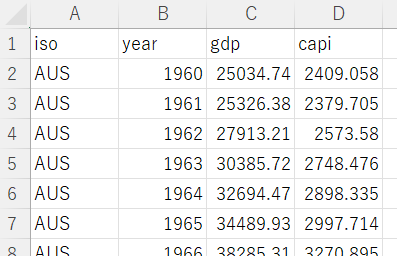So, I will conbine this GDP data and Material productibity data.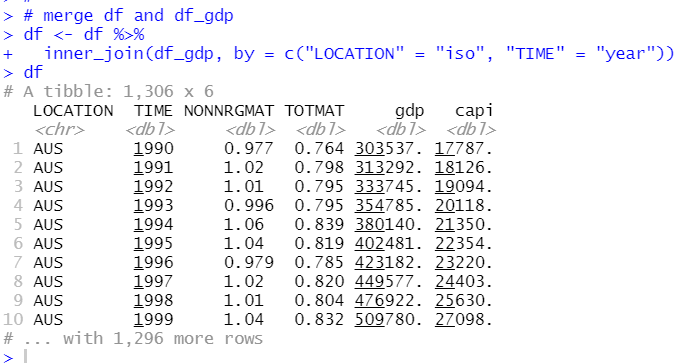let's see summary stats.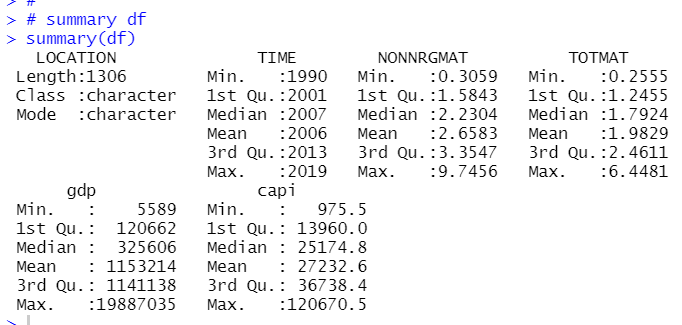That's it.
I will investigater further in next post.

Thank you!

The next post is